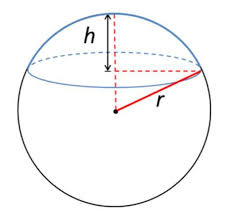## How to Calculate the Radius, Height and Curved Surface Area of a Spherical Cap | The Calculator EncyclopediaThe image above is a spherical cap.

To compute the curved surface area of a spherical cap requires two essential parameters which are the radius of the base of the cap (a) and the height (h).

The formula for calculating the curved surface area of the spherical cap:

A = π(a² + h²)

Where;
A = Curved surface area of the spherical cap
a = Radius of the base of the cap
h = Height of the spherical cap

Let’s solve an example;
Find the curved surface area of a spherical cap with radius of the base 7 cm and the height of 13 cm.

This implies that;
a = Radius of the base of the cap = 7 cm
h = Height of the spherical cap = 13 cm

A = π(a² + h²)
A =  π(7² + 13²)
A =  π(49 + 169)
A =  π(218)
A = 684.867

Therefore, the curved surface area of the spherical cap is 684.867 cm².

Calculating the Radius of the base of a Spherical Cap using the Curved Surface Area of the Spherical Cap and the Height.

a = √A – πh2 / π

Where;
A = Curved surface area of the spherical cap
a = Radius of the base of the cap
h = Height of the spherical cap

Let’s solve an example;
Find the radius of the base of a spherical cap when the curved surface area of the spherical cap is 300 cm2 and a height of 7 cm.

This implies that;
A = Curved surface area of the spherical cap = 300 cm2
h = Height of the spherical cap = 7 cm

a = √A – πh2 / π
a = √300 – 3.142 x 72 / π
a = √300 – 3.142 x 49 / π
a = √300 – 153.958 / π
a = √146.042 / π
a = √46.48
a = 6.82

Therefore, the radius of the base of the cap is 6.82 cm.